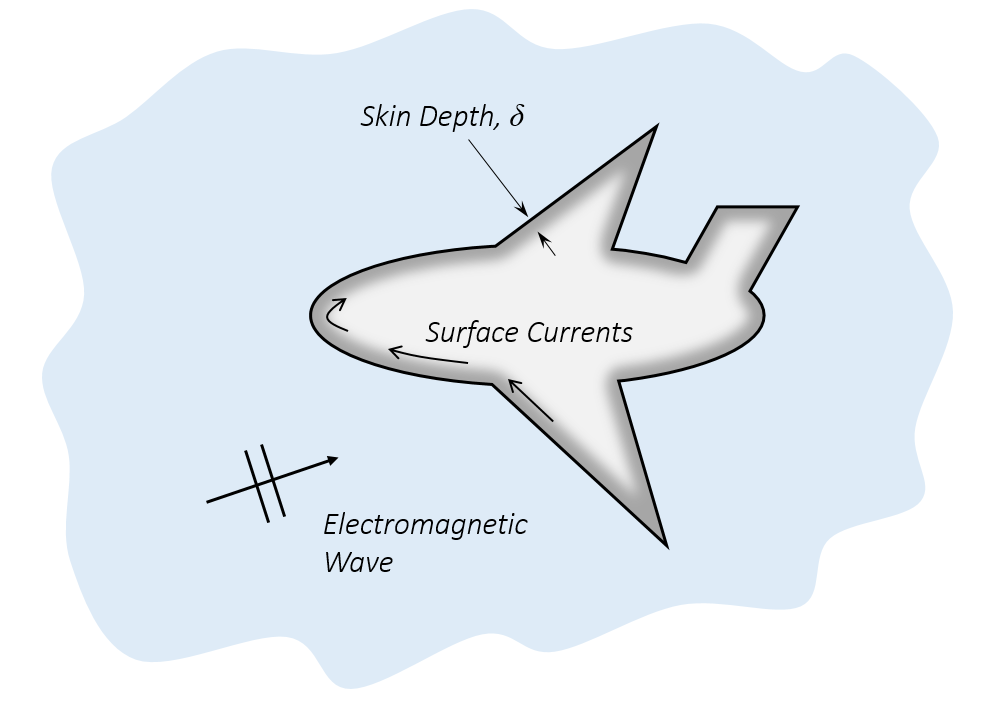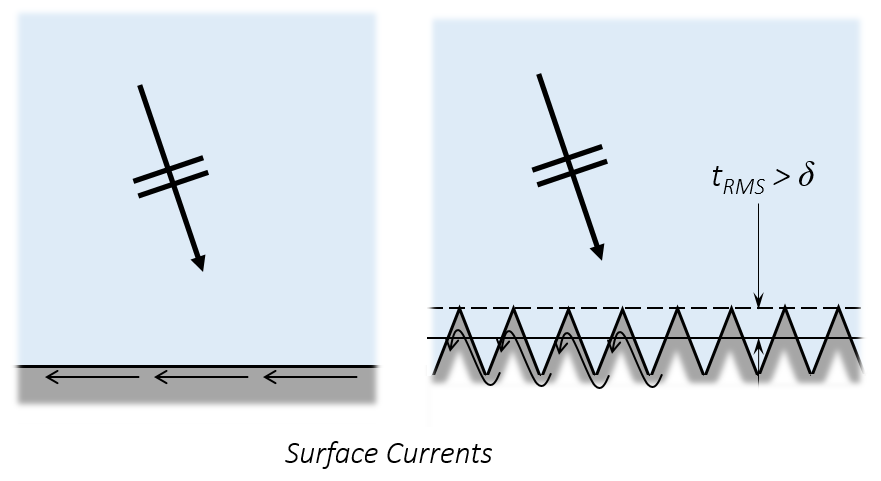# 模拟电磁波问题中的金属对象

2015年 5月 14日

### 什么是金属？

\nabla \times \left( \mu_r^{-1} \nabla \times \mathbf{E} \right) – {-\frac{\omega^2}{c_0^2}} \left( \epsilon_r -\frac{i \sigma}{\omega \epsilon_0} \right) \mathbf{E}= 0

\delta=\left[ \operatorname{Re} \left( \sqrt{i \omega \mu_0 \mu_r (\sigma + i \omega \epsilon_0 \epsilon_r)} \right) \right] ^{-1}

\delta=\sqrt{\frac{2}{\omega \mu_0 \mu_r \sigma }}

### 阻抗边界条件### 过渡边界条件

TBC 会将材料属性及膜厚度作为输入项，并通过膜厚度及切向阻抗来计算阻抗。这些可以用于将膜两侧表面的电流关联起来。也就是说，TBC 会造成所传输电场的下降。### 增加表面粗糙度### 完美电导体边界条件

PEC 边界是一种无损耗表面；能够 100% 反射入射波。该边界条件可以满足许多模拟需求，可以用在模型开发的早期阶段。有时，查看您的设备在零材料损耗下的表现也会很有意思。

### 小结

#### 评论 (2)

##### 留言##### 夏平 汤
2023-04-18##### hao huang
2023-04-19 COMSOL 员工

Email: support@comsol.com